# DRILL STRING DRAG DEFINITION AND CALCULATIONS

## TORQUE AND DRAG

### Definition Of The Drill String Drag

#### Consider an object of weight W is resting on a horizontal plane.In order to slide the object a force Ft must be exerted to overcome the force of friction Ff between the object and the plane surface.

The magnitude of the frictional force is
where,
Ff = frictional force, lb
µ = Friction Coefficient, dimensionless is  a constant that depends on the roughness of the object  and  plane  surface, Determined experimentally.
W = normal force acting perpendicular to the surface, lb

Therefore, the force Ft (Drag Force) required to slide the object should be slightly greater than Ff (friction Force)

### Now  consider  a  section  of  Drill Pipes  in  a wellbore inclined at an angle I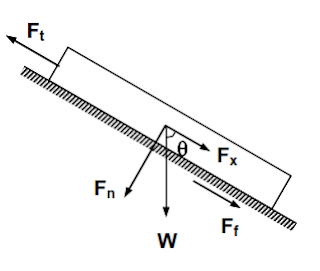Drill pipe section
1- The buoyed weight of the Drill Pipes W is acting vertically downward and can be resolved into two forces
• Fn (normal force acting perpendicular to the hole)
• Fx (the weight component acting parallel to the hole axis).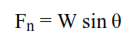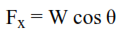2- The frictional or drag force Ff  is the normal force times the Friction Coefficient.

The frictional force or drag always acts in the opposite direction of the pulling force Ft. If the Drill Pipes is lowered into the hole, the frictional force will act in a direction opposite to that.

### Drill String Drag Calculations In Deviated Hole

In a curved section of the wellbore where inclination  and  azimuth  change  across the section, the calculation of the drag force is more difficult.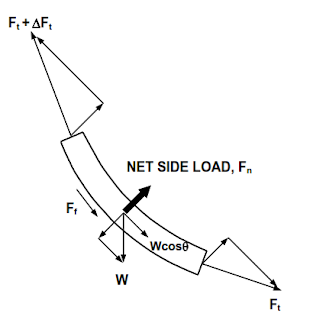This drawing shows that the forces acting on a short slightly curved element of the Drill String.

1- The weight of the element

2- Normal forces  from  the  tension  forces  Ft   and Ft + delta Ft as a result of the change in the angle of inclination and the azimuth of the wellbore.

The magnitude of the resultant normal force can be estimated by an   equation presented Johancsik, Friesen and Dawson,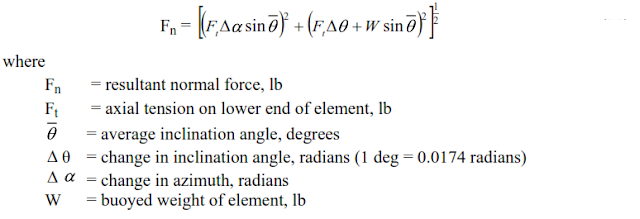If the Drill String is moved up hole (or down hole) while being rotated as in back reaming,
the drag force is calculated by using the following equation,

### What Is The Relation Between Critical Hole Angle And Drill String drag force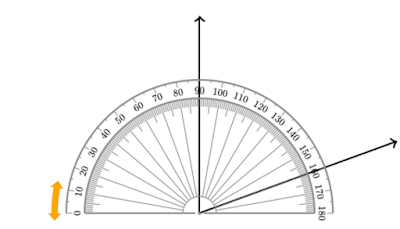The critical Angle

When the weight component of the drill string in the direction of the hole axis is equal to the drag force resisting downhole movement, the drill string is not able to slide down hole by its own weight and when drilling in the sliding mode the drill string will require pushing with pipe higher in the hole. The angle at which down hole movement becomes impossible is called the critical hole angle,

### Example On The Drill String Drag Calculations

Given:

#### Well bore

9-5/8” 40# casing at 5000 ft, Total Depth = 10,000 ft, Hole Size = 8-1/2”, Kick off Point = 5000 ft
Build Rate = 10 deg/100 ft, End of Build at = 5600 ft, Tangent Section = 5600 to 10,000
Inclination across tangent section = 60 deg, Azimuth = 0 deg north (surface to 5000 ft)
Azimuth is changed at the rate of 5 deg / 100 ft from 5000 ft to 5600 ft
Azimuth = 30 deg from 5600 ft to 10,000 ft
Mud Weight = 90 pcf, Coefficient of friction = 0.25 in open hole and 0.2 in casing.

#### Drill String

Drill pipe 5” OD, 5.276”ID, 19.5# normal weight 20.89# adjusted weight
Drill Collars 7” OD, 2.25” ID, 117.42#, 400 ft long

With the drill string at bottom, calculate:

a) The tension at surface required to move the drill string up hole
b) Total drag force when moving string up hole
c) The hook load when the string is off bottom and not moving

The first step is to divide the drill string into small elements from bottom to top as shown in below Table. The drill collars are considered as one element since there is no change in inclination or azimuth across them. The drill pipe in the tangent section from 9600’ – 5600’ is also taken as one element for the same reason. The drill pipe in the curved section from 5600 to 5000 is divided into twelve 50-ft elements because the inclination and azimuth change across the curved section. The last element is the vertical section from 5000’ to surface. The entries in the columns are defined as follows:
Column # 1: element sequence number

Column # 2: depth of the bottom of the element

Column # 3: element length in feet

Column # 4: angle of inclination in degrees at the bottom of the element

Column # 5: average angle of inclination across the element, or angle at bottom of
element plus angle at top of element divided by 2.

Column # 6: change in angle of inclination across the element in radians, or angle at
bottom of element minus angle at top of element. Multiply answer by
0.0174 to convert from degrees to radians.

Column # 7: azimuth at bottom of element in degrees.

Column # 8: change in azimuth in radians, or azimuth at bottom minus azimuth at
top multiplied by 0.0174.

Column # 9: buoyed weight of the element which is the weight in air times the
buoyancy factor. For drill pipe use the actual or adjusted weight per
foot.

Column # 10: tension force Ft at the bottom of the element. In this case since the drill string is off bottom Ft = 0 for element # 1. If the string is on bottom then Ft is a compressive force equal to the weight on bit.
Column # 11: normal force Fn calculated by using Eq (32)
Column # 12:
ΔFt calculated by below Eq.
Column # 13: tension at the top of the element and is equal to the value of Col# 11
plus the value of Col# 12.

Calculations for Element # 1

Column # 1: Element #1 consists of the entire drill collars which lie across the tangent section.

Column # 2: Depth at the bottom of drill collars is 10000 ft.

Column # 3: Length of drill collars is 400 ft.

Column # 4: Inclination at bottom of drill collars is 60 deg.

Column # 5: Average inclination is 60 deg because it is across tangent section, i.e. inclination at bottom of drill collars is same as at top.

Column # 6: Change in inclination in zero 60-60=0

Column # 7: Azimuth at bottom of drill collars is 30 deg.

Column # 8: Change in azimuth is zero because azimuths at top and bottom of drill
collars are the same.

Column # 9: buoyed weight of drill collars Buoyancy factor = 490-90 /49 = 0.815
W = 400 x 117.42 x 0.815 = 38278 lb

Column # 10: The tension at the bottom of the drill collars is zero because the drill string is off bottom.

Column # 11: The normal force Fn is calculated by this Eq
Fn = 33149 lb

Column # 12: Δ F is calculated by above Eq
ΔFt = Wcosθ + µFn = 38278 cos60 + 0.25 x 33149 = 27426 lb

Column # 13:
Ft+ Δ Ft = 0 + 27426 = 27426 lb
This is the tension at the top of the drill collars which is equal to the tension Ft
at the bottom of element # 2

Calculations for Element # 2

at the bottom of element # 2

Column # 1: This element is the bottom 4000 ft of 5” DP across the tangent section.

Column # 2: Depth of bottom end is at 9600 ft.

Column # 3: Length is 4000 ft.

Column # 4: Inclination angle across tangent section is 60 deg.

Column # 5: Average inclination angle is 60 deg.

Column # 6: No change in inclination angle.

Column # 7: Azimuth is constant at 60 deg.

Column # 8: No change in azimuth, zero

Column # 9: Weight of DP is,
W = 4000 x 20.89 x 0.815 = 68101 lb

Column # 10: This is the tension at the bottom of the element which is equal to the tension at the top of the previous element.

Column # 11: The normal force is from above Eq

Fn = 58977 lb

Column # 12: Δ F from Eq
Δ Ft = Wcosθ + µFn
Δ Ft = 68101 x cos60 + 0.25 x 58977 = 48794 lb

Column # 13: Tension at top of element is
Ft + Δ F = 27426 + 48794 = 76220 lb

Calculations for Element # 3

Column # 1: This is a 50-ft element of 5” DP at the bottom of the build section. The inclination angle at the lower end is 60 deg and at the upper end is 55 deg (build rate of 10 deg/100 or 5 deg per 50 ft). The azimuth at lower end is 30 deg N and at upper end is 27.5 deg N (azimuth is changed at rate of 5 deg/100 ft or 2.5 deg per 50 ft.)

Column # 2: Depth of lower end of element is 5600’ – end of tangent section.

Column # 3: length of element is 50 ft.

Column # 4: Inclination at bottom of element is 60 deg. Inclination at top of element is 55 deg.

Column # 5: Average inclination is
= 60+55/2 = 57.5 deg
Column # 6: Change in inclination angle is 60-55 = 5 degrees
5 x 0.01744 = 0.0872 radians

Column # 7: Azimuth at lower end is 30 deg.

Column # 8: Change in azimuth is  Azimuth at lower end – Azimuth at upper end
30 – 27.5 = 2.5 deg
2.5 x 0.01744 = 0.0436 radians

Column # 9: W = 50 x 20.89 x 0.815 = 851 lb

Column # 10: Tension at bottom of element is equal to tension at top of previous element 76220 lb.

Column # 11: Fn = 7879 lb

Column # 12:
Δ Ft = 851cos57.5 + 0.25 x 7879 = 2427 lb

Column # 13: Tension at top of element is
2427 + 76220 = 78647 lb

Calculations for elements 4 through 14 are done in the same manner.

Calculations for Element # 15

Column # 1: This is the last element. It is the DP in the vertical section from surface to the kick off point at 5000 ft.
Column # 2: Depth of lower end is at 5000 ft.

Column # 3: Length is 5000 ft.

Columns # 4 through 8: All values are zero because the pipe is vertical

Column # 9:
W = 5000 x 20.89 x 0.815 = 85126 lb

Column # 10: Tension at bottom end is 110427 lb
Column # 11: Fn = 0
The normal force is zero because the pipe is vertical and is not lying on the hole.

Column # 12:
ΔFt=  85126 x cos0 + 0.25 x 0 = 85126 x 1.0 + 0  = 85126 lb

Column # 13: Tension at the surface is
85126 + 110427 = 195,553 lb

Therefore, it requires a pull of 195,553 lb to move the pipe uphole. The Landmark torque and drag software (WELL PLAN) gave a value of 193, 500 lb.

b) The total drag force is the sum of all drag forces generated by all elements, or
F μ (Total Drag Force)

This is the sum of all normal forces times the friction coefficient. The sum of all normal forces is obtained by adding all values of F in Col # 11 which is 195176 lb.
Therefore,

Total Drag = 195176 x 0.25 = 48794 lb

c) The hook load when the pipe is static is
195553 - 48794 = 146,759 lb

The hook load is also equal to